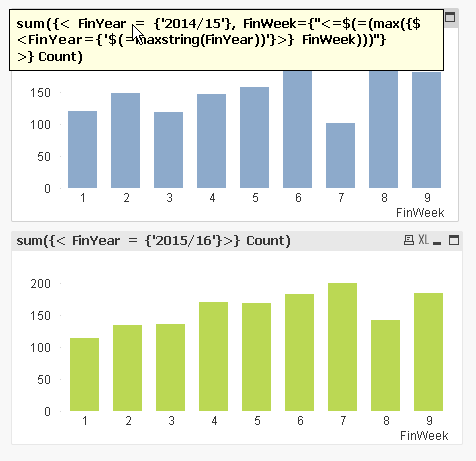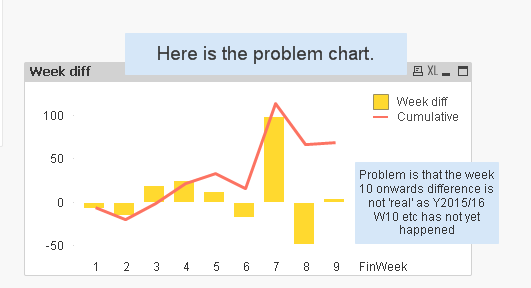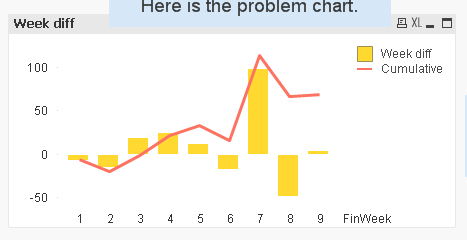# QlikView App Dev

Discussion Board for collaboration related to QlikView App Development.

Announcements
cancel
Showing results for
Did you mean:Not applicable

## Stop calc at current year max week number

Hello experts,

I want to compare data from two distinct years, but only to the latest week from the current year.

So if I have 9 weeks of data in the current year I want to stop the calculation at week 9 in each year.

I've attached a Qvw to explain more fully.

Hopefully this is self explanatory.

Cheers.

1 Solution

Accepted SolutionsMVP

I think the expression for 2014/15 should be (look at the image)

sum({< FinYear = {'2014/15'}, FinWeek={"<=\$(=(max({\$ <FinYear={'\$(=maxstring(FinYear))'}>} FinWeek)))"} >} Count)

Replace the same expression in the difference7 RepliesSpecialist III

Hi Jeff,

Is this what you are looking for?Calculation stops at week 9.

I am attaching the qvw as well.

ThanksMVP

I think the expression for 2014/15 should be (look at the image)

sum({< FinYear = {'2014/15'}, FinWeek={"<=\$(=(max({\$ <FinYear={'\$(=maxstring(FinYear))'}>} FinWeek)))"} >} Count)

Replace the same expression in the differenceMVP

May be this as your expression:

If(sum({< FinYear = {'2015/16'}>} Count) > 0, sum({< FinYear = {'2015/16'}>} Count) - sum({< FinYear = {'2014/15'}>} Count))MVP

or this should also work:

If(Len(Trim(Avg({< FinYear = {'2015/16'}>} Count))) > 0, sum({< FinYear = {'2015/16'}>} Count) - sum({< FinYear = {'2014/15'}>} Count))Not applicable
Author

Hi MaxGro - thanks for this very much appreciated - just what I was looking for.Not applicable
Author

Thanks Sinan not quite the way I needed this time, thanks for the pointers in any case.

J.Not applicable
Author

Thanks Sunny - both examples work - and the principles you employed will be helpful as I develop the overall dash.

Cheers

J.